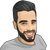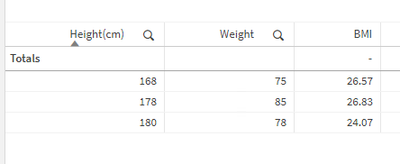Announcements
Applications are open for the 2024 Qlik Luminary Program. Apply by December 15 here.
cancel
Showing results for
Did you mean:Contributor III

## How to Calculate BMI

Hi I have height ( in centimetes)  and Weight  (in kgs)columns, From this How to calculate BMI

 Height(cm) Weight(kg) 168 75 180 78 178 85
Labels (3)

• ### Set Analysis

1 Solution

Accepted SolutionsPartner - Creator III

If you want to add the BMI calculation to the client, You can add a measure to the straight table with the following formula:

``(Weight/[Height(cm)]/[Height(cm)]) * 10000``

Result:Eli.Partner - Creator III

If you want to add the BMI calculation to the client, You can add a measure to the straight table with the following formula:

``(Weight/[Height(cm)]/[Height(cm)]) * 10000``

Result:Eli.Tags
Community Browser